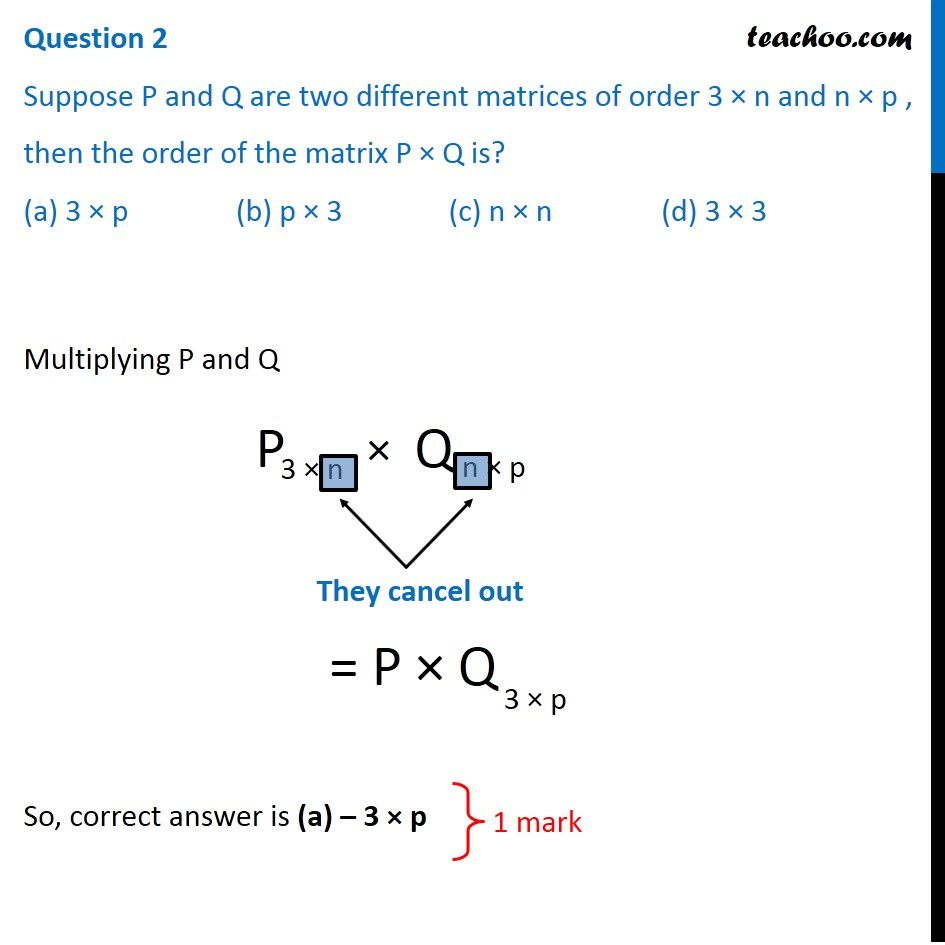## (a) 3 × p            (b) p × 3            (c) n × n            (d) 3 × 31. Class 12
2. Solutions of Sample Papers and Past Year Papers - for Class 12 Boards
3. CBSE Class 12 Sample Paper for 2020 Boards

Transcript

Question 2 Suppose P and Q are two different matrices of order 3 × n and n × p , then the order of the matrix P × Q is? (a) 3 × p (b) p × 3 (c) n × n (d) 3 × 3 Multiplying P and Q They cancel out = P × Q So, correct answer is (a) – 3 × p 1 mark

CBSE Class 12 Sample Paper for 2020 Boards

Class 12
Solutions of Sample Papers and Past Year Papers - for Class 12 Boards